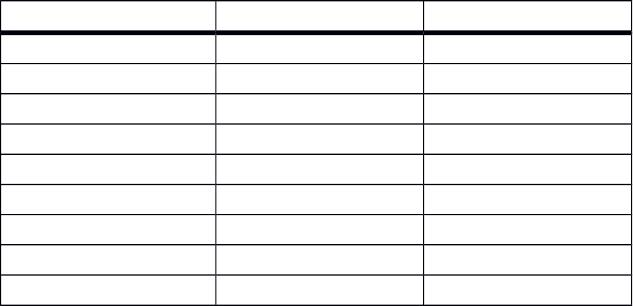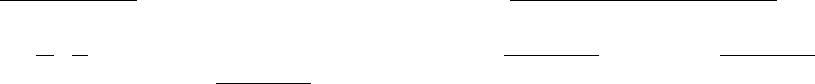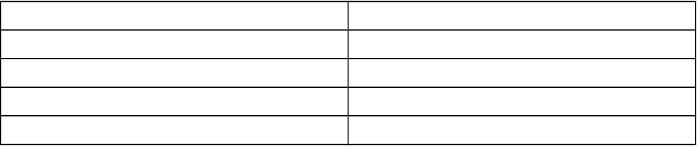Type
Quiz
Book Title
Basic Marketing Research: Using Microsoft Excel Data Analysis 3rd Edition
ISBN 13
978-0135078228

### 978-0135078228 Chapter 9 Lecture Note

June 7, 2019
CHAPTER 9
Determining Sample Size and the Sample Plan
LEARNING OBJECTIVES
To become familiar with the basic concepts involved in sampling
To learn how to calculate the minimum sample size needed to achieve a predetermined level
of accuracy
To understand the difference between “probability” and “nonprobability” sampling plans
To become acquainted with the specifics of four probability and four nonprobability
sampling plans
CHAPTER OUTLINE
Basic Concepts in Samples and Sampling
Determining Size of a Sample
The Accuracy of a Sample
Formula to Determine Sample Accuracy
How to Calculate Sample Size When Estimating a Percentage
Variability: p times q
Level of Confidence: z
Desired Accuracy: e
Explaining the Logic of Our Sample Size Formula
How to Calculate Sample Size When Estimating a Mean
The Effects of Incidence Rate and Nonresponse on Sample Size
1
Using the XL Data Analyst to Calculate Sample Size
How to Select a Representative Sample
Probability Sampling Methods
Simple Random Sampling
Using the XL Data Analyst to Generate Random Numbers
Systematic Sampling
Cluster Sampling
Stratified Sampling
Nonprobability Sampling Methods
Convenience Samples
Judgment Samples
Referral Samples
Quota Samples
Online Sampling Techniques
KEY TERMS
Accuracy of a sample
Area sampling
Census
Cluster sampling
Completed interviews
Completion rate
Proportionate sample size
Proportionate stratified sample
Quota sample
Random digit dialing
Random numbers technique
Random online intercept sampling
2
Confidence interval formula for sample
size
Convenience sample
Demographic incidence
Desired accuracy
Disproportionate sample size
Geographic incidence
Incidence
Invitation online sampling
Judgment sample
Level of confidence
Nonprobability sampling method
One-step area sample
Online panel sampling
Population
Probability sample methods
Product incidence
Random sample
Random starting point
Referral sample
Sample
Sample frame
Sample frame error
Sampling error
Simple random sampling
Skip interval
Stratified sampling
Surrogate measure
Systematic sampling
Table of random numbers
Two-step area sample
Variability
Weighted average
Working phone rate
TEACHING SUGGESTIONS
31. National opinion polls tend to be around 1,000–1,200 in sample size, and this can be easily
verified. Have students do background research on opinion polls (perhaps there is a political
campaign underway) and bring their findings to class on the sample size and reported errors.
The findings illustrate that these companies are using the sample size for a percentage
formula with p and q equal to 50 percent and with a 95 percent level of confidence.
2. Figure 10.1 illustrates visually how large sample sizes fail to add to the accuracy of a survey.
Because the graph is difficult to read with precision, consider using the following table to
illustrate to students how little additional accuracy is gained with increases in the sample
size, especially with large samples.
Sample Size 95% Accuracy Accuracy Increase
100 9.8% -
250 6.2% 3.6%
500 4.4% 1.8%
750 3.6% 0.8%
1,000 3.1% 0.5%
1,250 2.8% 0.3%
1,500 2.5% 0.3%
1,750 2.3% 0.2%
2,000 2.2% 0.1%
3. The confidence interval approach to sample size involves several statistical concepts.
Although they are described in the chapter, it is necessary to review them thoroughly in class.
Students may memorize the sample size determination formula, but it is important that they
comprehend the factors in the formulas, namely, (1) the amount of variability believed to be
in the population, (2) the desired accuracy, and (3) the level of confidence required in
estimates of the population values.
4. Students do not easily grasp the concept of a sampling distribution. If you have a class of,
say, 30 students, you can pair them up and simulate 15 different samples. Have each pair give
its average age (other variables might be: hours carried this term, hours worked per week,
minutes to commute to campus), and plot all of the sample means in a distribution. If you
select a variable where students are quite similar (e.g., age), the sampling distribution will be
quite compact, but if you use a variable where students are dissimilar (e.g. distance of home
town away from campus), the sampling distribution will be much less compact.
5. Use the 95 percent level of confidence in sample size determination examples. The 1.96 z
value is about 2, and students have an easier time following the squaring computations than
they do with the 2.58 z value for the 99 percent level of confidence.
6. The sample size determination function in the XL Data Analyst provides sensitivity analysis
tables where the estimated “p” and the allowable error are varied slightly. This feature is
4
provided so users do not need to run the analysis several times. It shows how sensitive
sample size is to variations in p of ±5% and ±10% and variations in the allowable error of
±.5% and ±1%. An extension of this feature is to add a cost factor (such as x dollars per
respondent) and to show how the sensitivity estimates affect the cost of the sample.
7. The equiprobable aspects of a random sample can be demonstrated in a number of different
ways. Here are two examples:
Some Internet sites are devoted to lotteries and gambling. Although we do not have a
specific one in mind, you might consider assigning a student or a team of students to
search out one or more that lists the lottery numbers selected over time, perhaps for your
state if you have a state lottery. Have students calculate the percent of times each number
was drawn and comment on whether or not they have found the random selection process
used to embody equal probability in all cases.
Put 3 x 5 index cards with students’ names in a hat or a box and have a series of actual
blind draw samples. Replace the drawn names to the population pool after each sample.
Maintain a record of how often each student’s name is selected.
8. The greater efficiency of systematic sampling over simple random sampling can be
demonstrated with a class exercise. Identify two groups of students and give each a copy of
the same page from the telephone book. Tell the first group to select 10 household names
using a table of random numbers or random numbers generated via a spreadsheet program
such as Microsoft Excel (simple random sampling), and tell the second group to select 10
names using systematic sampling. The second group should finish before the first group.
9. A class exercise that illustrates basic differences between various sample methods is to use a
data set with 10 or fewer variables listed in labeled columns and containing 200 or more
respondents (an Excel or other spreadsheet printout will work). Break the class into several
teams and give specific instructions for each team to draw 30 respondents and calculate the
percentage distribution or mean of the variables. Each team should be assigned a different
sampling method (convenience, simple random sampling, etc.). Have students time their
teams or raise their hands when they have completed the work. Student teams using
nonprobability sampling (except quota) will finish first, while teams using the more tedious
probability methods such as stratified simple random sampling will finish last. However,
nonprobability sampling method teams will have findings different from the true percentage
distributions and means, although probability sampling method teams will have findings
close to the actuals. (Note: Instructors will have to provide a table or list of random numbers
to teams needing them. Such tables can be generated by the random numbers feature of the
XL Data Analyst.)
10. When learning about the skip interval used in systematic sampling, students sometimes ask
how to determine the population size when a directory or phone book is used. In the absence
of a precise number, the size is usually estimated by multiplying the approximate number of
names on each page by the number of pages. Some adjustments may need to be made for
5multiple listings such as children’s phone, discontinued phones, or areas of “white space” in
the directory.
11. Some students may have difficulty understanding the weighted average calculations in
stratified sampling. It may be necessary to illustrate how the mean changes with different
stratum configurations. Here are some comparisons than can be used to demonstrate the
effects of three different weighted average situations.
Stratum Mean Estimated Population Mean
A B 50%/50% 40%/60%
10%/90%
5 8 6.5 6.8
7.7
12. Students should come to realize that the success of quota sampling is greatly dependent on a
prior knowledge of the population’s characteristics. One way to facilitate this understanding
is to ask students what quota characteristics should be used in the following two cases.
Case One. Kellogg’s wants to know the reactions of parents to a new children’s cereal called,
“Cheery-Os.”
Case Two. Proctor & Gamble wants the reactions of potential buyers of its new hair rinse
called, “Gentle Care.”
With case one, the quota characteristics would be: (1) parents (percent female versus male),
(2) marital status (percent married versus separated), and (3) age of youngest child (percent
4, 5, 6, etc.). With case two, however, the target market is not identified well other than it is
13. Instructors can use the random numbers function of the XL Data Analyst to illustrate the
nature of random numbers. Use it to generate a large number of random numbers, then
use Excel average and standard deviation functions to compute these values of each row and
each column.
In theory, the average of any random number table row or any column should be
approximately equal to the average of any other row or column. The standard deviations
should be approximately equal as well.
14. When students work with a familiar population, they are better able to apply sample methods.
Ask how the full-time students in your university would be sampled using each sample
method described in the chapter. For example, where would they station interviewers for a
convenience sample? How would they set up clusters or strata using student characteristics?
15. Here is a table the summarizes the differences between probability sampling methods and